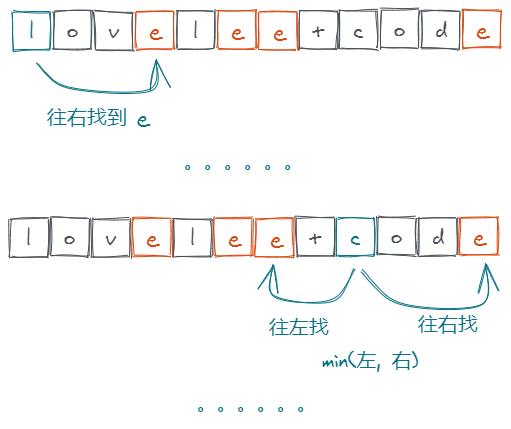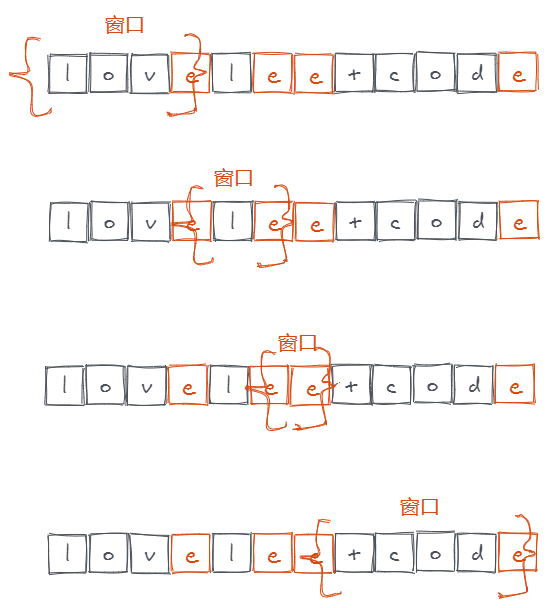我是如何把简单题目做成困难的？

|

解法 1：中心扩展法

思路

• 从当前下标出发，分别向左、右两个方向去寻找目标字符 C
• 只在一个方向找到的话，直接计算字符距离。
• 两个方向都找到的话，取两个距离的最小值。复杂度分析

• 时间复杂度：$O(N^2)$，N 为 S 的长度。
• 空间复杂度：$O(1)$。

JavaScript Code

解法 2：空间换时间

复杂度分析

• 时间复杂度：$O(N*K)$，N 是 S 的长度，K 是字符 C 在字符串中出现的次数。由于 $K <= N$。因此时间上一定是优于上面的解法的。
• 空间复杂度：$O(K)$，K 为字符 C 出现的次数，这是记录字符 C 出现下标的辅助数组消耗的空间。

JavaScript Code

解法 3：贪心

思路

1. 从左往右 遍历字符串 S，用一个数组 left 记录每个字符 左侧 出现的最后一个 C 字符的下标；
2. 从右往左 遍历字符串 S，用一个数组 right 记录每个字符 右侧 出现的最后一个 C 字符的下标；
3. 然后同时遍历这两个数组，计算距离最小值。

1. 字符左侧没有出现过 C 字符
2. i - left > right - i (i 为当前字符下标，left 为字符左侧最近的 C 下标，right 为字符右侧最近的 C 下标)

复杂度分析

• 时间复杂度：$O(N)$，N 是 S 的长度。
• 空间复杂度：$O(1)$。

JavaScript Code

JavaScript Code

Python Code:

解法 4：窗口

思路

C 看成分界线，将 S 划分成一个个窗口。然后对每个窗口进行遍历，分别计算每个字符到窗口边界的距离最小值，并在遍历的过程中更新窗口信息即可。复杂度分析

• 时间复杂度：$O(N)$，N 是 S 的长度。
• 空间复杂度：$O(1)$。

JavaScript Code